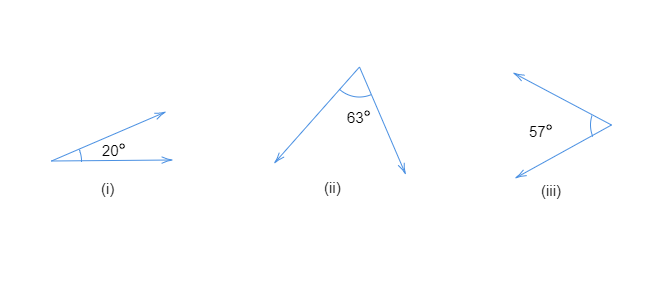# Find the complement of each of the following angles:"

To do:

We have to find the complement of each of the given angles.

Solution:

Two angles are said to be complementary if the sum of their measures is $90^{\circ}$.

If two angles are complementary, each angle is said to complement of the other angle.

Complement of an angle $x$ is $90^{\circ}-x$.

Therefore,

(i) Complement of $20^{\circ}$

$= 90^{\circ}-20^{\circ}$

$=70^{\circ}$

(ii) Complement of $63^{\circ}$

$= 90^{\circ}-63^{\circ}$

$=27^{\circ}$

(iii) Complement of $57^{\circ}$

$= 90^{\circ}-57^{\circ}$

$=33^{\circ}$